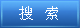说明：双击或选中下面任意单词，将显示该词的音标、读音、翻译等；选中中文或多个词，将显示翻译。 您的位置：首页 -> 句库 -> 折旧费用 1. Depreciation Expense-Furniture and Fixtures折旧费用-家具及装修 2. Depreciation Expense-Land Improvements折旧费用-土地改良 3. Some expenses such as depreciation expense, amortization expense and depletion expense are non-cash expenses. 像折旧费用、摊销费用、递耗费用之类的费用属于非现金费用。 4. The straight-line method allocates an equal portion of depreciation expense to each period of the asset's useful life. 直线折旧法在资产使用年限内将等额折旧费用分配到每一年度。 5. The cost of depreciation and expired insurance may be recorded as adjustments at the end of the accounting period. 折旧费用和已耗保险费于会计期末在调整分录中记录。 6. It is "gross" because it does not deduct any charge for the depreciation of capital used up in the course of this production. 它由于未扣除该生产过程中资本损耗的折旧费用而被称为“总值”。 7. The Comparison and Analysis of Fixed Assets Depreciation Costs between Standards of Accountancy and Tax Law; 固定资产折旧费用在会计准则与税法中的比较分析 8. an allowance for loss due to depreciation.弥补因折旧而损失的费用。 9. an amount periodically charged to expense or against revenue in compensation for depreciation of property. 定期承担的财产折旧的补偿费用。 10. Annual depreciation expense then is computed by applying this accelerated depreciation rate to the undepreciated cost (current book value) of the asset. 年折旧费就用这个加速折旧率乘以待折旧成本(即当时的账面净值)得出。 11. Depreciation Expense = Remaining Book Value X Accelerated Depreciation Rate折旧费=剩余账面净值×加速折旧率 12. Depreciation Expense = Remaining Book Value * Accelerated Depreciation Rate折旧费=剩余账面净值×加速折旧率 13. For example, depreciation on machinery used in production is properly considered part of overhead, while depreciation on cars used by the sales staff is not; the latter cost is a selling cost. 如机器设备的折旧是制造费用,销售部门所用汽车的折旧就不是制造费用而是销售成本。 14. cost of reproducing physical property minus various allowances (especially depreciation).再生产现货资产的费用减去各种补助费（尤其是折旧）。 15. The journal entry to record depreciation expense consists of a debit to Depreciation Expense and a credit to Accumulated Depreciation. 记录折旧的会计分录包括借记折旧费账户和贷记累计折旧账户。 16. The delivery truck in our example has an estimated life of 5 years, so the depreciation expense each year is 1/5, or 2%, of the depreciable amount. 我们例中的运输卡车有5年预计使用年限,所以每年的折旧费是可供折旧金额的1／5,或2％。 17. Understand the concept of" Depreciation" and the assumptions used to calculate depreciation. 理解“折旧”概念和用于计算折旧的假设。 18. the expense of maintaining property (e.g., paying property taxes and utilities and insurance); it does not include depreciation or the cost of financing or income taxes. 为保持财产所必须支付的费用（如：财产税、使用费和保险费）；不包括折旧或金融业或所得税。 ©2011 dictall.com